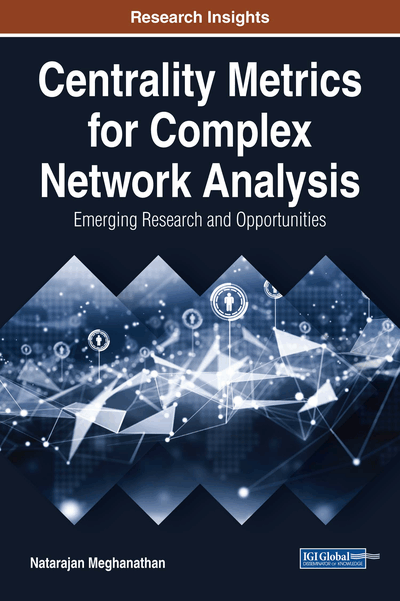# Centrality-Based Assortativity Analysis of Complex Network Graphs

Copyright: © 2018 |Pages: 12
DOI: 10.4018/978-1-5225-3802-8.ch003

## Abstract

In this chapter, the author analyzes the assortativity of real-world networks based on centrality metrics (such as eigenvector centrality, betweenness centrality, and closeness centrality) other than degree centrality. They seek to evaluate the levels of assortativity (assortative, dissortative, neutral) observed for real-world networks with respect to the different centrality metrics and assess the similarity in these levels. The author observes real-world networks are more likely to be neutral (neither assortative nor dissortative) with respect to both R-DEG and BWC, and more likely to be assortative with respect to EVC and CLC. They observe the chances of a real-world network to be dissortative with respect to these centrality metrics to be very minimal. The author also assesses the extent to which they can use the assortativity index (A.Index) values obtained with a computationally light centrality metric to rank the networks in lieu of the A.Index values obtained with a computationally heavy centrality metric.
Chapter Preview
Top

## 2. Assortativity Index Formulation And Example

The assortative index (A. Index) of a real-world network with respect to a centrality metric is computed as the Pearson’s correlation coefficient of the centrality values of the end vertices of the edges. The Pearson’s product-moment correlation coefficient between two sets of vertices X and Y (whose entries represent the centrality values for one or more vertices with respect to a particular metric C) is calculated as follows. The jth element in the sets X and Y is denoted by xj and yj respectively. Letand(calculated as in formulation-1 below) be respectively the average values for the centrality metric of interest (C) among the vertices constituting the sets X and Y.

## Complete Chapter List

Search this Book:
Reset